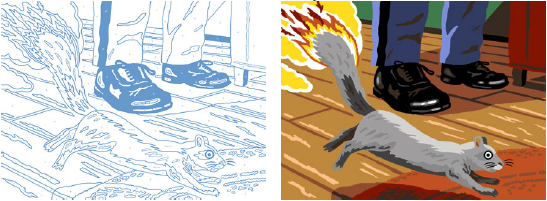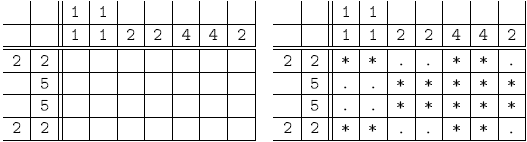## Day 2, Problem 3: Paint by Numbers

Years ago, there was a really bad craft/hobby called paint-by-numbers: you were given a line drawing, with numbers in each enclosed region, and the number corresponded to a particular colour. An example is shown below:The problem you have to solve is much more linear, in a way.

You will be given an n-by-m grid (1 ≤ n, m ≤ 32) which you will "colour" in with either a dot ('.') or a star ('*').

Of course, the grid will not be specified in the usual paint-by-numbers way, since this would be too easy.

Instead, you will you have to infer which cells are blank and which contain a star. The only information you will be given are a collection of n + m sequences of numbers, one sequence for each row and column. The sequence will indicate the size of each continuous block of stars. There must be at least one dot between two consecutive blocks of stars.

An example is shown below (which is supposed to look fish-like):You may notice that some paint-by-number patterns are not uniquely solvable. For this problem, you may assume that any solution which satisfies the specification is correct.

### Input

Input consists of a total of n + m + 2 lines.

The first line of the test case consists of an integer n (1 ≤ n ≤ 32), the number of rows.
The second line consists of an integer m (1 ≤ m ≤ 32), the number of columns.

On the next n lines, there will be sequences which describe each of the n rows (from top to bottom). Each line will contain some positive integers, with a space between adjacent integers, and the sequence will end with the integer 0.

On the next m lines describe the m columns (from left to right), the same format as the rows are specified.

### Output

Output consists of n lines, each line composed of m characters, where each character is either a dot ('.') or a star ('*').

### Sample Input 1

```4 7 2 2 0 5 0 5 0 2 2 0 1 1 0 1 1 0 2 0 2 0 4 0 4 0 2 0```

### Sample Output 1

```**..**. ..***** ..***** **..**.```

### Sample Input 2

```4 4 2 1 0 3 0 3 0 1 1 0 4 0 3 0 3 0 1 0```

### Sample Output 2

```**.* ***. ***. *.*.```

Point Value: 30 (partial)
Time Limit: 2.00s
Memory Limit: 16M